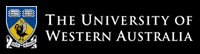School of Computer Science and Software Engineering

# School of Computer Science and Software Engineering

## CITS4406 Problem Solving and Programming

#### Labsheet 2: Review Excercises and Program Testing

This labsheet is in two parts: a number of review exercises taken from the text that reinforce concepts from the lectures; and an important problem-solving technique, motivated by its use in solving a larger-sized problem representative of the types of problems that can be solved with computers.

#### Exercises

1. Jack says that he will not bother with analysis and design but will proceed directly to coding his programs. Why is that a bad idea?

2. x = 'hello' will assign the string "hello" to the varialble x. How does one include an apostrophe as a character within a string literal? Try it and then try to include a double-quote character within a string?

3. Include both apostrophe and double -quotes in one string literal.

4. How would you print two lines using a single print command?

5. How would you print one line using two print commands i.e. split one line between two print commands?

6. How does a Python programmer concatenate two string literals? Try it.

7. Assign two variables simultaneously and then swap them. Now try the same two steps with three variables.

8. Write a Python function that calculates the factorial of a number.  Inside the function, Prompt the user to give the number.

9.Python has a math module cotaining useful mathematical functions. Import the math module and use it to calculate the square root of a float. Try out other math functions as well such as using it to calculate the factorial of an integer.

10. Modify the futval.py program so that the number of years for the investment is also a user input.

11.Write a Python function that takes an integer parameter N and prints all the odd numbers from 1 to N except those divisible by 5.

12. Try giving a float value to the above function and see what happens. Now modify the function such that when it is given a parameter of type float, it terminates and prints a message that the input must be an integer.

#### Problem Solving Technique: Morphological Analysis and Testing

In Chapter 2 of the text we learnt about the importance of the testing activity during software development.

In an introductory course it is likely that you will be responsible for testing your own programs as you develop them and before you submit them for assessment. In the software industry, and as part of other research and development tasks that may involve programming, it is often the case that separate individuals or teams of people are involved in writing the programs and testing them. It is common for much of the testing to be undertaken as "Black Box Testing", which means that the testers try running the program on a range of inputs without looking in detail at the code. (Often the code is not in an easily readable form).

In today's lab you will imagine yourself in a testing team, testing someone else's program.

tri.py is a Python program that attempts to classify a triangle on the basis of the lengths of its sides. (Note that three lengths can form a triangle if they are all positive, and the longest is smaller than the sum of the other two.) Your task is to devise and carry out a Black Box testing process on the code. You need to propose a range of inputs, and expected answers, and see if the program gives the correct answers. You may assume that all three arguments to tri are positive. Do not examine the code itself.

The objective is to determine if the program is correctly implemented. If it is not you should report on what sorts of input cause erroneous output. Show your report to the lab demonstrator when you are done.

Hint: Copy & paste the program into a file called tri.py. Recall from Lab 1 how to run a Python program from a .py file using IDLE. First run the program on a few simple inputs to see how it behaves. When you understand what it should be doing, try and devise a complete classification for the different sorts of inputs that could be presented to the program. Make sure that you test each sort of input at least once.

The different types of inputs can be listed in a table (like the one given below) and all classes of test inputs and expected outputs can be filled in:

 Case No. Description Input Values Expected Output 1. - - - 2. - - - 3. - - -

Making a complete classification of the sorts of inputs that can be given to a piece of software is an example of the general problem solving technique called Morphological Analysis. (Morphological means to do with the shape of something. You can read more about morphological analysis here).

# The University of Western Australia

## University information

CRICOS Code: 00126G